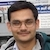# Inverted Glass Experiment | Fluid PressureBy

To show that air exerts pressure, science textbooks at lower classes describe an activity where a glass tumbler is filled to the brim with water, is covered by a cardboard and then inverted. The demo consists of seeing that the cardboard does not fall. The explanation is that air exerts force on the cardboard from below and this force is more than that exerted on the cardboard by the water. So the card is pushed up and does not fall. The present demo is a slight modification in this famous activity wherein the water is not filled up to the brim and then it becomes a demo for Boyle's law.

## Procedure

Take a glass tumbler and fill it up to about half with water. Put a plane cover on the glass. You can use a cardboard, or a plastic cover or any other plane cover. Hold the cover by pressing from top with one hand and invert the glass. Gently remove the hand from the cover. The cover does not fall and holds the water in the glass.

## Discussion

The upper portion of the glass contains air and hence presses the water down. The force on the cover due to water is $$A(P_1+ h\rho g)$$; where $$A$$ is the area in the upper portion of the glass, $$h$$ is the height of the water column and $$\rho$$ is the density of water. From the bottom the force is $$P_0A$$; where $$P_0$$ is the atmospheric pressure. The fact that the card does not fall tells that $$P_1 < P_0$$. How did $$P_1$$ become smaller than $$P_0$$? When you covered the glass, the air trapped was at the atmospheric pressure $$P_0$$. It is the same air that is now in the upper portion (provided you have not allowed air to leak out or leak in during inverting the glass) the volume of air should be the equal to $$V_\text{air}=V_\text{glass}-V_\text{water}$$. If both remain the same, the volume of air should remain the same. The temperature is anyway the same. So from $$PV=nRT$$, the pressure should remain the same, that is $$P_0$$.

But it does not remain $$P_0$$. It becomes less than $$P_0$$. In fact the cover goes slightly down when the glass is inverted. If it is a cardboard cover, it may buldge at the centre. If it is plastic cover, it goes slightly down and there is a water slice between the glass and the cover. This you can verify by slightly tapping the cover horizontally. You will find that the cover moves quite smoothly. This shows that it is not rubbing the glass surface. Here surface tension also has to play a role.

As the cover goes slightly down, the volume of air in the upper portion increases. This decreases in pressure according to the Boyle's law and the water column stays in equilibrium.

Sometimes few drops of water may go out while inverting. If air has not gotten into the glass in this period, the volume of air will increase and hence the pressure will decrease. Sometimes few bubbles of air also gets in. This happens when somewhat larger mass of water comes out during inversion. In such a case right amount of air will go in which can maintain the cardboard in equilibrium.

## Variants

Do the same with holes in the card. Water does not come out through the holes.

## Related

Subscribe to our channel